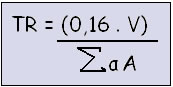Sound and Oscillation

# Reverberation time

The reverberation time is the amount of time the sound is audible at a given location.

We can consider that the reverberation time is the time when the sound is audible in a given place, which can be a classroom, an auditorium, a theater, a cinema, in short, any place that can be influenced by sound.

This question is very common in classrooms. This is because, in most cases, the buildings made to house schools are designed outside the architectural standards that allow for better sound distribution.

This affects both teachers and students, because the greater the distance between student-teacher, the greater the difficulty in making themselves heard, even if they try hard.

For the place to present sound audibility, the most important reference is the volume of the medium where the waves propagate.

Reverberation time is calculated by the Sabine equation, the father of modern acoustics. The formula is represented mathematically as:Where:
TR = reverberation time (seconds)
0.16 = constant
V = room volume (m 3 )
? = sum
α = absorption coefficient of materials
A = floor, wall and ceiling area (m 2 )